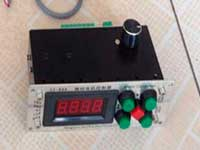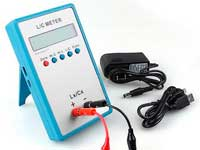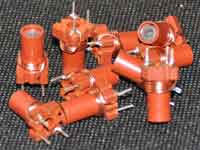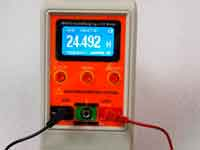The self-resonance frequencyIn the early days of the Age of Radio, it was discovered that the helix inductor is not an ideal inductor. At a certain frequency it is in the resonance mode even in the absence of external capacitance, and above this frequency the impedance of the coil is capacitive. To explain this phenomenon assumed that in addition to self-inductance real coil has a self-capacitance (conceivably between adjacent turns) and the coil is presented as a model of lumped RLC elements, where L — inductance, C — self-capacitance, and R takes into account the various losses in the coil. Such a model has only one resonant frequency, called the self-resonance frequency of the coil (SRF). This model became a classical model of the real coil in all textbooks.

Because the coil, in the majority of practical applications, is working at frequencies far below the SRF, the purpose of the designer is the providing this condition. The majority of engineers with this purpose tried to reduce this "inter-turn stray capacitance". In case, if the coil operates at frequencies close to SRF, as for example in a helical resonator or Tesla coils, RLC-model gives incorrect results, but in such cases, had developed an alternative calculation algorithms and all designers were satisfied and not really turned over in one's mind about the reasons for such inconsistencies.

Parallel resonant LC circuit

A parallel resonant circuit in electronics is used as the basis of frequency-selective networks. Real circuit elements have losses, and when we analyse the LC network we use a realistic model of the ideal lumped elements in which losses are taken into account by means of "virtual" serial resistances RL and RC.About Q-factor of RF inductance coilCalculation of Q-factor inductor is quite complex. Ultimately, the quality factor depends on many factors - the loss in the wires, in core,  in shield. Accurate accounting of theese all are very difficult. However, we can simplify the problem, if we take into account only the losses in the wire. Firstly, they are major contributors to the total amount of losses, and secondly estimate the quality factor of the coil we are interested under creating more high Q circuits. In this case, we are applying the special actions to minimize the losses by the way of implementing a ribbing core or an air-core inductance coil.

Is there, I ask, can there be, a more interesting study than that of alternating currents

Nicola Tesla

The self-capacitance of a single-layer air core solenoid

At high frequencies, the inductor would be correctly represented as a helical single-wire transmission line. As is known, such a structure is defined as the small inductances and capacitances distributed along the line length. When the length of this line is equal to λ / 4 (λ - wavelength of the electromagnetic wave in the coil), the line is in resonance mode and the frequency corresponding to this wavelength is called the self-resonant frequency of the coil. Coils rarely operate at frequencies close to the self-resonance or above. However, if this happens, such as in RF choke, the calculation of the coil is preferable to carry out in an RF electromagnetic simulators.

Simple calculation formulas of coil inductance

Formulas and numerical methods of calculation of inductance coils, which uses Coil32, you can look in the help section of the site, namely:

Coil Winding MachineUS \$187.53
The system consists of individual components including a controller, a stepping motor, a foot switch, power adapter and output shaft. This design makes it very easy for you to integrate the system into your own project.

LC Meter LC200AUS \$37.99
Measuring Capacitance Range:
0.01 pF - 100mF
Measuring Inductance Range:
0.001 uH - 100H
Measurement accuracy:1%.

RF InductorsUS \$6.43
Variable Ferrite Core Inductor coil set 3.5Turns 25-100MHZ

Auto Ranging LCR MeterUS \$63.07
Meter can measure 0.00pF to 100.00mF capacitance, 0.000uH to 100.00H inductance and 0.00? to 10.000M? resistance. Very suitable for small value capacitor and inductor measurement.